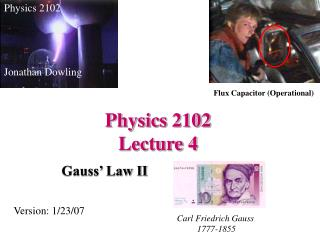DownloadDownload PresentationPhysics 2102 Lecture 4

# Physics 2102 Lecture 4

Download Presentation## Physics 2102 Lecture 4

- - - - - - - - - - - - - - - - - - - - - - - - - - - E N D - - - - - - - - - - - - - - - - - - - - - - - - - - -
##### Presentation Transcript

1. Physics 2102 Jonathan Dowling Physics 2102 Lecture 4 Flux Capacitor (Operational) Gauss’ Law II Version: 1/23/07 Carl Friedrich Gauss 1777-1855

2. HW and Exam Solutions • www.phys.lsu.edu/classes/spring2007/phys2102/Solutions/index.html • USERNAME: Phys2102 • Password: Solution1 • Both are: cAsE SenSiTivE!

3. Properties of conductors Inside a conductor in electrostatic equilibrium, the electric field is ZERO. Why? Because if the field is not zero, then charges inside the conductor would be moving. SO: charges in a conductor redistribute themselves wherever they are needed to make the field inside the conductor ZERO. Excess charges are always on the surface of the conductors.

4. -15 C Gauss’ Law: Example • A spherical conducting shell has an excess charge of +10 C. • A point charge of -15 C is located at center of the sphere. • Use Gauss’ Law to calculate the charge on inner and outer surface of sphere • (a) Inner: +15 C; outer: 0 • (b) Inner: 0; outer: +10 C • (c) Inner: +15 C; outer: -5 C R2 R1

5. -5 C +15C -15C Gauss’ Law: Example • Inside a conductor, E = 0 under static equilibrium! Otherwise electrons would keep moving! • Construct a Gaussian surface inside the metal as shown. (Does not have to be spherical!) • Since E = 0 inside the metal, flux through this surface = 0 • Gauss’ Law says total charge enclosed = 0 • Charge on inner surface = +15 C Since TOTAL charge on shell is +10 C, Charge on outer surface = +10 C - 15 C = -5 C!

6. Faraday’s Cage • Given a hollow conductor of arbitrary shape. Suppose an excess charge Q is placed on this conductor. Suppose the conductor is placed in an external electric field. How does the charge distribute itself on outer and inner surfaces? (a) Inner: Q/2; outer: Q/2 (b) Inner: 0; outer: Q (c) Inner: Q; outer: 0 • Choose any arbitrary surface inside the metal • Since E = 0, flux = 0 • Hence total charge enclosed = 0 • All charge goes on outer surface! Inside cavity is “shielded” from all external electric fields! “Faraday Cage effect”

7. More Properties of conductors We know the field inside the conductor is zero, and the excess charges are all on the surface. The charges produce an electric field outside the conductor. On the surface of conductors in electrostatic equilibrium, the electric field is always perpendicular to the surface. Why? Because if not, charges on the surface of the conductors would move with the electric field.

8. Charges in conductors • Consider a conducting shell, and a negative charge inside the shell. • Charges will be “induced” in the conductor to make the field inside the conductor zero. • Outside the shell, the field is the same as the field produced by a charge at the center!

9. Gauss’ Law: Example • Infinite INSULATING plane with uniform charge density s • E is NORMAL to plane • Construct Gaussian box as shown

10. Gauss’ Law: Example • Infinite CONDUCTING plane with uniform areal charge density s • E is NORMAL to plane • Construct Gaussian box as shown. • Note that E = 0 inside conductor For an insulator, E=s/2e0, and for a conductor, E=s/e0. Does the charge in an insulator produce a weaker field than in a conductor?

11. Q Q/2 Insulating and conducting planes Insulating plate: charge distributed homogeneously. Conducting plate: charge distributed on the outer surfaces.

12. + + + + + + + + + + + + E=0 Gauss’ Law: Example • Charged conductor of arbitrary shape: no symmetry; non-uniform charge density • What is the electric field near the surface where the local charge density is s? (a) s/e0 (b) Zero (c) s/2e0 THIS IS A GENERAL RESULT FOR CONDUCTORS!

13. +10 C E -15C E=k(15C)/r2 E=k(5C)/r2 E=0 r Electric fields with spherical symmetry: shell theorem A spherical shell has a charge of +10C and a point charge of –15C at the center. What is the electric field produced OUTSIDE the shell? If the shell is conducting: And if the shell is insulating? Charged Shells Behave Like a Point Charge of Total Charge “Q” at the Center Once Outside the Last Shell! Conducting

14. Summary: • Gauss’ law provides a very direct way to compute the electric flux. • In situations with symmetry, knowing the flux allows to compute the fields reasonably easily. • Field of an insulating plate: s/2e0, ; of a conducting plate: s/e0.. • Properties of conductors: field inside is zero; excess charges are always on the surface; field on the surface is perpendicular and E=s/e0.